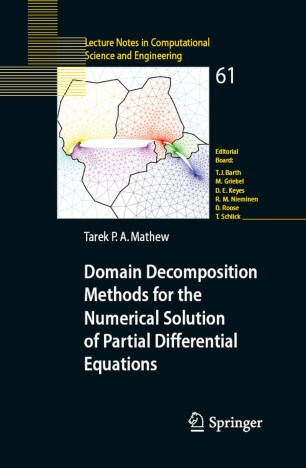# Domain Decomposition Methods for the Numerical Solution of Partial Differential Equations

• Authors
• Tarek Poonithara Abraham MathewBook

Part of the Lecture Notes in Computational Science and Engineering book series (LNCSE, volume 61)

1. Front Matter
Pages I-XIII
2. Pages 1-46
3. Pages 47-105
4. Pages 263-294
5. Pages 295-312
6. Pages 313-331
7. Pages 377-416
8. Pages 417-513
9. Pages 515-573
10. Pages 575-606
11. Pages 607-619
12. Pages 621-646
13. Pages 647-678
14. Pages 679-687
15. Pages 689-698
16. Pages 699-709
17. Back Matter
Pages 711-764

### Introduction

Domain decomposition methods are divide and conquer methods for the parallel and computational solution of partial differential equations of elliptic or parabolic type. They include iterative algorithms for solving the discretized equations, techniques for non-matching grid discretizations and techniques for heterogeneous approximations. This book serves as an introduction to this subject, with emphasis on matrix formulations. The topics studied include Schwarz, substructuring, Lagrange multiplier and least squares-control hybrid formulations, multilevel methods, non-self adjoint problems, parabolic equations, saddle point problems (Stokes, porous media and optimal control), non-matching grid
discretizations, heterogeneous models, fictitious domain methods, variational inequalities, maximum norm theory, eigenvalue problems, optimization problems and the Helmholtz scattering problem. Selected convergence theory is included.

### Keywords

algorithm algorithms differential equation finite elements linear algebra numerical analysis optimization parallelization partial differential equation partial differential equations scientific computing

Industry Sectors